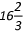Percentage is a favourite topic of examiners across competitive examinations. Most students skip statement-based questions on Percentages and fractions because they appear to be lengthy and time-consuming. However, a good hold on decimals, fractions and percentages provide you an extra edge over other candidates.
###### Basic Percentage Formula
1. A basic knowledge of fractions and percentages is essential to solve the advance level questions. E.g. 1/3 = 33% and so on.
2. An increase/ decrease of x % on a number n, yields (1+ x/100)n. E.g. an increase of 20 % on x makes it 1.2x, similarly a decrease of 33% on x makes it 0.66x.
###### Solved Percentage problems
Example 1: An increase of 30% in the price of oranges enables a man to buy 6 kg less for Rs. 300. Find the increased price per kg.
Solution: The statement implies that the man has 30% of Rs. 300 available to spend on 6 kgs now. 30% of 300 = 30/100 x 300 = 90
So, the increased price per kg is 90/6 = Rs. 15 per kg
###### Learning
• This question can be solved by forming equations and then solving for price and quantity. However, a careful analysis of the statement using the concept of percentage equips you to solve the problem in a fraction of seconds!
Example 2: The difference between the marked price and the selling price on an item is same as the difference between the cost price and the selling price. Find the profit percent if the discount of% is offered on the item.
Solution: The statement can be written in the equation form as,
Marked price - Selling price = Selling Price - Cost Price. Let us call this Equation (1). Now, the discount offered is% which is nothing but 1/6 in fraction form. We know, Discount = Marked price - Selling Price. So, if the marked price is Rs. 6, then the Selling Price is Rs. 5. In that case, Cost Price will be equal to Rs. 4 according to equation (1). Hence, the profit percentage = Cost price - Selling price/ Cost price × 100. Substituting the values, profit percentage = ¼ × 100 = 25%.
###### Learning
• In most of the questions, assuming a value for the unknown and then solving the equation can prove to be the real catch. In this question, since, the denominator is 6, marked price should essentially be taken as a multiple of 6. In other cases, using 100 as the value of the unknown can easily crack the problem.
Take Test :
Know where you stand in Percentage topic, take this test now.

Example 3: Let A & B be two spheres such that the surface area of B is 300% greater than that of A. The volume of A is found to be k% lower than that of B, find the value of k.
1. 85.5
2. 92.5
3. 87.5
4. 90.5
Solution: Surface area of B = Surface area of A + 3(100%) Surface area of A
Which implies, Surface area of B = 4 Surface area of A (Equation 1)We know, Surface area of sphere = 4πr2 & the volume of the sphere = 4/3 πr3So, with respect to A and B,Sa: Sb = 1:4ra: rb = 1:2Va: Vb= 1:8. Therefore, 1 - (Va /Vb) = 7/8. According to the question, Va = kVb. Hence, k= 7/8 = 87.5%. Thus, option C is the correct answer.
###### Learning
This is an actual exam question. A combined application of Geometry and percentage change is favourite among examiners.
The key in such questions is
• To be aware of the formula of the Surface area and Volume for various figures viz. Sphere, hemisphere, cone, cuboid, etc.
• Be careful of the squaring or cubing of the change term. Most of the students commit a mistake in this step in a hurry to solve the question
Take Test :